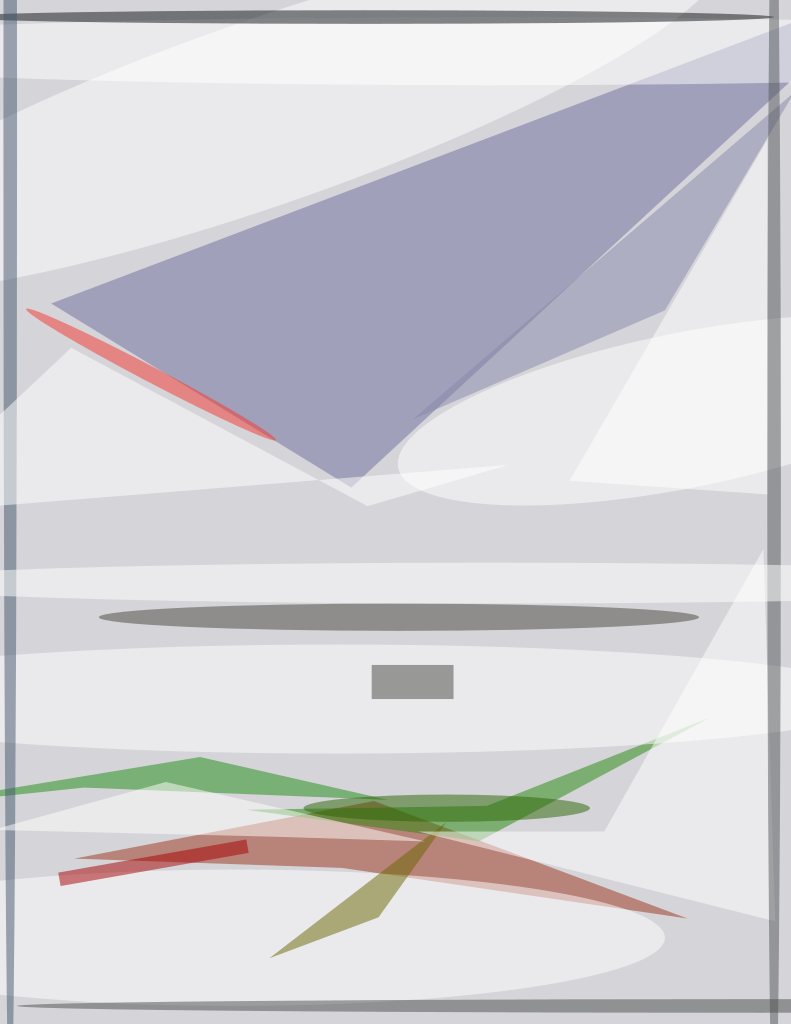The phenomenon of flapping wing flight in nature has been studied for centuries. Recently, flapping flight for unmanned aerial vehicle (UAV) applications has become of interest. Flapping wing flight offers many potential advantages over traditional fixed- and rotary-wing aircraft. Fixed-wing UAVs have the advantages of long range and endurance, and high payload capabilities; however, they require high forward flight speeds and most configurations cannot hover, which makes them difficult to control in confined spaces.

Conversely, rotary-wing UAVs are highly maneuverable, can fly at lower forward speeds, and can hover, but generally have lower endurance times and are louder than fixed-wing UAVs due to high rotor tip speeds. The goal of using flapping-wing UAVs (FWUAVs) is to bridge the gap between fixed- and rotary-wing UAVs. FWUAVs have the potential to fly at lower airspeeds than fixed-wing aircraft, and most have the ability to hover, which enables FWUAVs to be flown in confined spaces. Compared to rotorcraft, FWUAVs tend to be quieter since the wing flapping speed is generally much slower than a rotor’s rotation. This combination of maneuverability, hover capability, and stealthiness makes FWUAVs a potential choice for use in confined spaces.Figure 2. (top) Wing Chord Shapes along the span, and (bottom) two-dimensional airfoil shapes from the 3D wing scatter plots. This project is based on the bird-inspired FWUAV called the RoboRaven, which was designed and built at the University of Maryland (Figure 1). Bird-inspired flight is based on the forward posture of birds, where a forward velocity is required to maintain lift and the flapping motion is in a roughly vertical plane with respect to the forward velocity. RoboRaven has a wingspan of 0.9 m and a maximum chord length of ~0.3 m. The wings can move independently of one another, driven by two separate servos, which rotate the wings at approximately 3–4 Hz. In flight, the RoboRaven cruises at approximately 6.7 m/s, which is in the flight regime of Reynolds number ~120,000.

The goal is to improve upon the accuracy of existing FWUAV aerodynamic models to be used in the conceptual design process. FWUAV design is currently a sequential trial and error process, where engineers iterate through many different designs until reaching a desirable configuration. Aerodynamic models that can accurately predict flight forces enable designers to run through many iterations of design prior to building any prototypes.

The Blade Element Momentum Theory (BEMT) aerodynamic analysis was created using a combination of two aerodynamic theories: Blade Element Theory (BET) and momentum theory. In BET, the wing of a UAV is discretized across the entire span into chordwise “slices.” Each of these slices experiences the flight forces of lift, drag, and thrust (in differential form). Because the wing is three-dimensional, each slice is really a small section with a width in the spanwise direction; however, it is treated as an airfoil with infinite span. Once all the differential forces have been calculated at each slice, integration is performed along the entire span to calculate the total forces on the wing.

Compared to BET, momentum theory is a much more simplified approach to calculating aerodynamic forces. In momentum theory, the momentum change of moving air deflected off a wing is used to calculate lift and thrust. When a wing is placed at an angle of attack, α, with respect to the forward velocity, U, it will deflect air downward at a velocity, w, called induced velocity. Induced velocity is ultimately used in momentum theory to calculate lift and thrust.

For a flapping wing, momentum theory analyzes the wings as a “partial actuator disk,” where the disk’s size is determined by the swept area of the wing flapping when viewing the UAV’s frontal area. The momentum change of the air moving across this partial disk area is used to calculate thrust. Thus, in momentum theory, the entire wing flapping motion is represented by the partial disk area, as opposed to discretizing the wing along the span as in BET.

In both BET and momentum theory, there is one unknown variable, induced velocity, in the equations used for thrust calculation. When BET and momentum theory are used separately, induced velocity is either approximated using a function or is assigned a constant value as defined by the individual performing the aerodynamic analysis. BEMT solves this problem by combining the two equations for BET and momentum theory in order to solve for downwash velocity. Once downwash velocity is calculated, it is used in the BET analysis at each spanwise “slice” and forces are calculated.

This project accomplishes the goal of calculating the lift coefficient based on wing shape through the use of Digital Image Correlation (DIC) wing deformation scatter plots. These scatter plots are simple 3D representations of the wing, taken directly from DIC images of an FWUAV flapping in a wind tunnel. Once the 3D wing shape scatter plots have been obtained from DIC images, it is necessary to calculate the lift coefficient to be used in BET portion of the BEMT code (Figure 2). The experimental phase of this project was completed entirely via computer modeling in MATLAB.

This work was done by Justin Alexander Yang of the Army Research Laboratory. ARL-0161